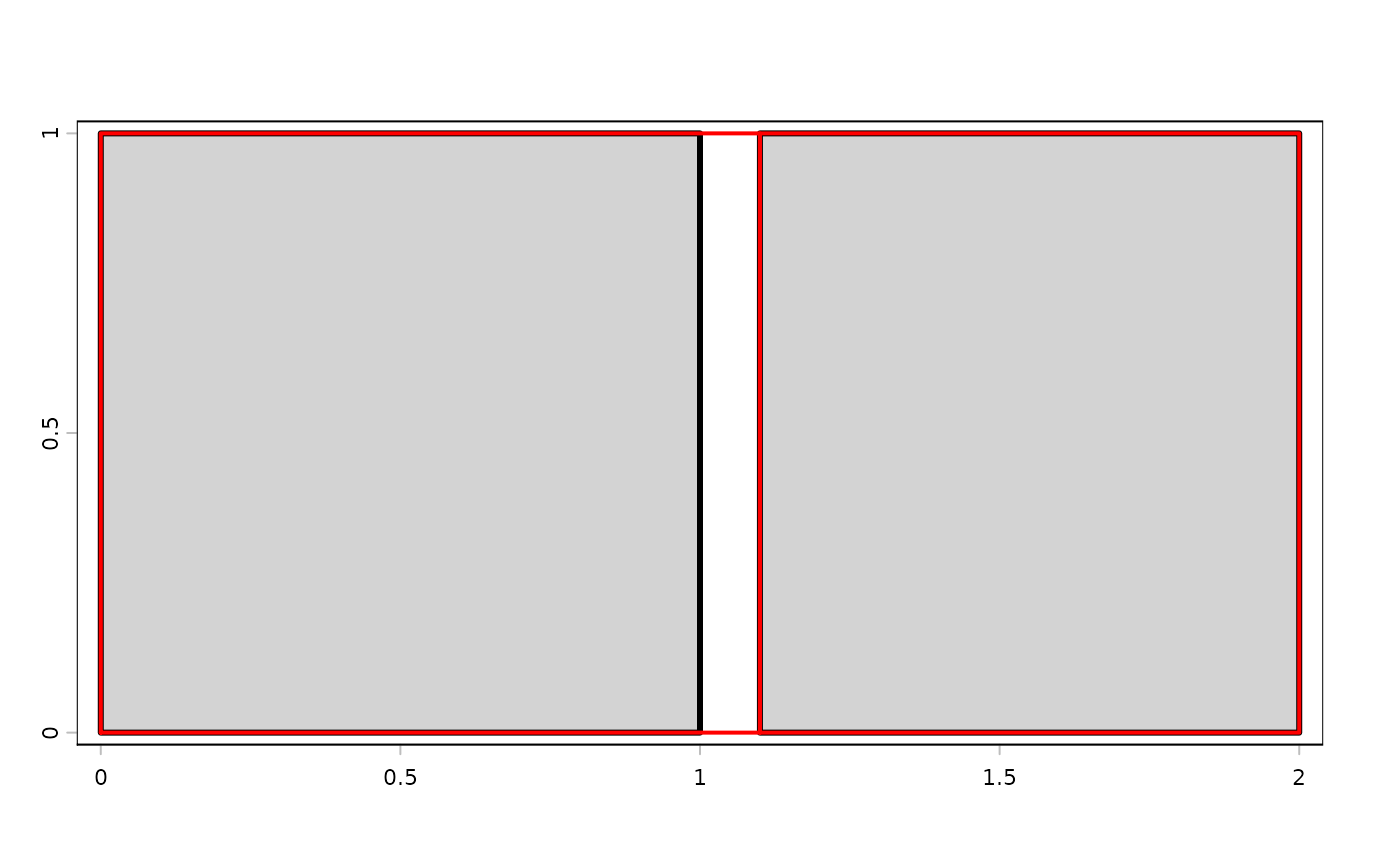makeNodes create nodes on lines

mergeLines connect lines to form polygons

removeDupNodes removes duplicate nodes in geometries and optionally rounds the coordinates

emptyGeoms returns the indices of empty (null) geometries

snap makes boundaries of geometries identical if they are very close to each other.

# S4 method for SpatVector
mergeLines(x)
# S4 method for SpatVector
snap(x, y=NULL, tolerance)
# S4 method for SpatVector
removeDupNodes(x, digits = -1)
# S4 method for SpatVector
makeNodes(x)

## Arguments

x

SpatVector of lines or polygons

y

SpatVector of lines or polygons to snap to. If NULL snapping is to the other geometries in x

tolerance

numeric. Snapping tolerance (distance between geometries)

digits

numeric. Number of digits used in rounding. Ignored if < 0

## Value

SpatVector

sharedPaths, gaps, simplifyGeom

## Examples

p1 <- as.polygons(ext(0,1,0,1))
p2 <- as.polygons(ext(1.1,2,0,1))

p <- rbind(p1, p2)

y <- snap(p, tol=.15)
plot(p, lwd=3, col="light gray")
lines(y, col="red", lwd=2)